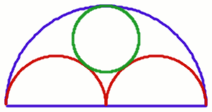#### You may also like### Diophantine N-tuples

Can you explain why a sequence of operations always gives you perfect squares?### DOTS Division

Take any pair of two digit numbers x=ab and y=cd where, without loss of generality, ab > cd . Form two 4 digit numbers r=abcd and s=cdab and calculate: {r^2 - s^2} /{x^2 - y^2}.### Sixational

The nth term of a sequence is given by the formula n^3 + 11n . Find the first four terms of the sequence given by this formula and the first term of the sequence which is bigger than one million. Prove that all terms of the sequence are divisible by 6.

# Three Four Five

##### Age 14 to 16Challenge LevelAs shown in the diagram, two semi-circles (each of radius $1/2$) touch each other, and a semi-circle of radius 1 touches both of them. Find the radius of the circle which touches all three semi-circles, and find a 3-4-5 triangle in the diagram.